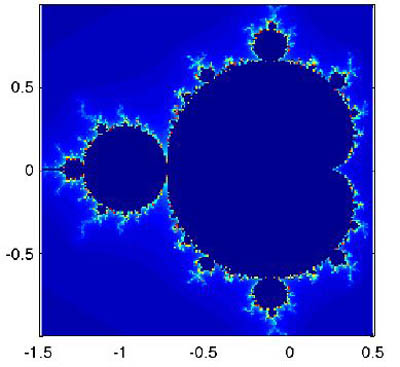18.S997 | Fall 2011 | Undergraduate

# Introduction To MATLAB Programming

## Fractals and ChaosGenerating fractals is a comprehensive way to utilize MATLAB®’s programming loops and logical expressions.

A fractal is a geometric figure that can be subdivided into parts that are mathematically similar to the whole. You will be asked to plot the Mandelbrot fractal, and effectively practice constructing `while` loops, which terminate based on a known and specified condition. You will also learn how to use commands that help you terminate the loop prematurely and otherwise modify the execution of the loop.

In this unit, you will also use comparative and logical operations to evaluate expressions and create a truth matrix composed of zeroes and ones. The truth matrix will be useful for logical indexing, which can be used in situations that require that certain elements of a list be separated from the rest of the elements based on a certain characteristic.